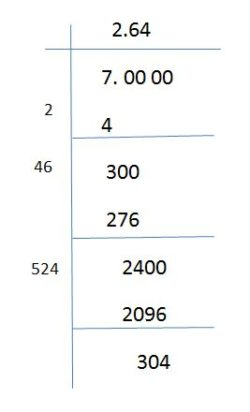# Square Root of 7

To find the square root of the given number, we need to know if it is a perfect square or not. Number 7 is not a perfect square. Therefore, we have to find the square root of 7 using the long division method. Mathematics is the science of numbers. Aryabhata was the first great mathematician of ancient India. In 1956 he introduced the method of finding the square root of a number. However, the square root sign was invented by Christoff Rudolff in the year 1525. A number, when multiplied by itself, gives the original number which is called as the square root of the number.

## Root 7 Value

Before finding the value of root 7, it is important to know what is perfect and non-perfect square. Any perfect square number ends with 1, 4, 5, 6, 9 in the unit place. Any number that ends with 2, 3, 7, 8 at the unit place is called non-perfect square. Check out the square root formula given by BYJU’S to find the square root of numbers.

Example: Let’s consider number 7

• 7 is a prime number
• It has no square factor

The square root of the non-perfect square can be found by any of the following methods:

• Using binomial theorem
• Using calculus
• Long division method

To find the square root of 7 we can use the long division method which is simple and easy.Therefore, the value of the square root of 7 is 2.64.

At BYJU’S, India’s best math teachers conduct math classes. They keep it interactive and interesting using videos and 3D graphics images to explain the concepts easily. Hence, students don’t lose interest in the subject and keep improving their skills by participating in the practice test and quiz.

Stay tuned with BYJU’S – The Learning App to learn more maths-related articles and also watch engaging videos.

Test your Knowledge on Square Root of 7